DATA MINING
Desktop Survival Guide
by Graham Williams# Overview

Support vector machines were introduced by Vapnik (1979, 1998). Their use has become widespread because of their sound theoretical foundations and demonstrated good results in practise. SVMs are based on the idea of structural risk minimisation (SRM).

We can understand the idea best as a binary classification problem, predicting two classes as -1 and 1. The idea is to find the best hyperplane separating the two classes in the training dataset. The best hyperplane is the on that maximises the margin between the two classes--it is the sum of the distances from the hyperplane to the closest positive and negative correctly classified samples. The number of miss-classifications is used to penalise the measure.

The hyperplane can be found in the original dataset (and this is referred to as linear SVMs) or it can be found in a higher-diemnsional space by transforming the dataset into a representation having more dimensions (input variables) than the original dataset (referred to as nonlinear SVMs). Mapping the dataset, in this way, into a higher dimensional space, and then reducing the problem to a linear problem, provides a simple solution.

Computational requirements for SVM are significant.

A kernel is a function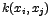which takes two entities (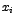and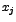and computes a scalar.

In choosing a kernel: the Gaussian kernel is a good choice when only the smoothness of the data can be assumed.

The main choice then is the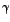, the kernel width for SVMs with Gaussian kernel.

The Gaussian kernel is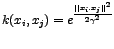. Here, the numerator is the squared 2-norm of the two vectors.